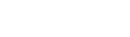# What Is The First Law Of Thermodynamics?

The first law of thermodynamics states that the change in internal energy of the system (ΔU) is equal to the amount of heat provided to the system (Q) minus the amount of work done by the system (W)

What happens when you switch on a lamp? The electrical energy coming through the wires is converted into light. When you crank up the ignition of your vehicle, the energy stored in the gasoline sets you in motion. All the daily activities that you do happen because of the calories that you eat in your food. Energy is converted from one form to another all the time! We see it happening at every moment of our conscious life.

In the realm of physics, there is a law for everything that happens in the world. The law that governs this phenomenon is the law of conservation of energy. The law states that energy can neither be created nor destroyed. It can only change from one form to another.

Thermodynamics is a branch of physics that deals with heat energy and its relationship with work and other forms of energy. The first law of thermodynamics is a version of the law of conservation of energy that relates to heat energy. The laws of thermodynamics define how work, heat, and energy affect a system. A system is any region in the Universe that is finitely bounded, across which energy is transferred. Everything outside this boundary is its surroundings.

Recommended Video for you:

## What Is The First Law Of Thermodynamics?

The first law of thermodynamics states that the change in internal energy of the system () is equal to the amount of heat provided to the system () minus the amount of work done by the system ():In some places, you can find it as:The difference between the two equations is that in the first one, W is work done by the system, whereas in the second one, it is the work done on the system. If the system does some work, then the first equation can be used, but if work is done on the system, then the second equation can be used.

Also Read: What Is The Second Law Of Thermodynamics?

## Why Are There Different Equations?

Although the law remains the same, the equations vary depending on the events occurring in the system. The first equation with a -W was defined by physicists dealing with internal combustion engines. In their case, the heat provided to the system expands the gas, which moves the piston or “does some work.” It was the equation that was used by Clausius, the scientist who first stated the law in its full form.

Chemists use the second equation with a +W because they mostly deal with systems where a process happens when work is done on the gases. This equation is part of the IUPAC convention and is the most commonly used equation today.

Also Read: What Is The Third Law Of Thermodynamics?

## Examples Of The First Law Of Thermodynamics

### Internal Combustion Engine

The classic example used to explain the first law of thermodynamics is the internal combustion engine. In an IC engine, a spark ignition combusts a mixture of air and gasoline. The combustion causes the gases inside the engine to expand. This expansion pushes the piston outwards, thus moving a vehicle forward, which is mechanical energy. In the process, the engine also gets heated up and loses some energy as heat energy.

If we put this in the equation, the change in internal energy (or) is the energy stored in the fuel. Q is the heat provided to the engine by the spark plug. Thus, W is the resulting movement (mechanical energy) and the heat (losses).

### Melting Ice Cube

Keep an ice cube on a plate and leave it. In a few minutes, it will melt, converting to water. The ice absorbs heat from the surrounding air, the air becomes cool, and the ice changes into water. In this case, the state of the matter is changing due to the absorption of heat. The internal energy (or) of ice is increasing as it absorbs energy (Q) from the surrounding air. As the process continues, the water turns into vapor if it is left for too long. The internal energy of the system (water) keeps changing, as it is open to a surrounding containing heat. Thus, the first law of thermodynamics governs the flow of energy.

## History Of The First Law Of Thermodynamics

The need to define such laws was first felt by humans when they invented the first engine. It became imperative to establish a relationship between heat and work. They wanted to increase the industrial output, so it was essential to define and improve the efficiency of the heat engines.

It took about half a century and inputs from many physicists to come up with the first law. Several scientists made statements that were close to this law. However, it was first stated in full by Rudolf Clausius and William Rankine in the year 1850.

What is the Second Law of Thermodynamics?

What is the Third Law of Thermodynamics?

How well do you understand the article above!

Can you answer a few questions based on the article you just read?References (click to expand)